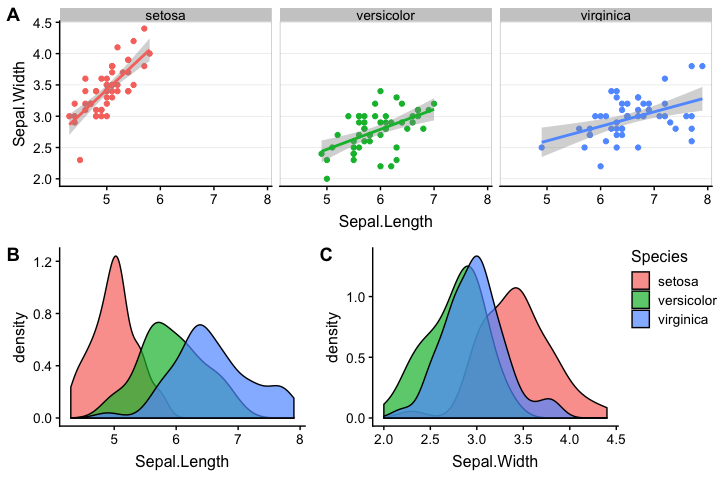# Shared legends

#### 2019-01-08

This vignette demonstrates how to make compound plots with a shared legend.

# down-sampled diamonds data set
dsamp <- diamonds[sample(nrow(diamonds), 1000), ]

# Make three plots.
# We set left and right margins to 0 to remove unnecessary spacing in the
# final plot arrangement.
p1 <- qplot(carat, price, data=dsamp, colour=clarity) +
theme(plot.margin = unit(c(6,0,6,0), "pt"))
p2 <- qplot(depth, price, data=dsamp, colour=clarity) +
theme(plot.margin = unit(c(6,0,6,0), "pt")) + ylab("")
p3 <- qplot(color, price, data=dsamp, colour=clarity) +
theme(plot.margin = unit(c(6,0,6,0), "pt")) + ylab("")

# arrange the three plots in a single row
prow <- plot_grid( p1 + theme(legend.position="none"),
p2 + theme(legend.position="none"),
p3 + theme(legend.position="none"),
align = 'vh',
labels = c("A", "B", "C"),
hjust = -1,
nrow = 1
)
prow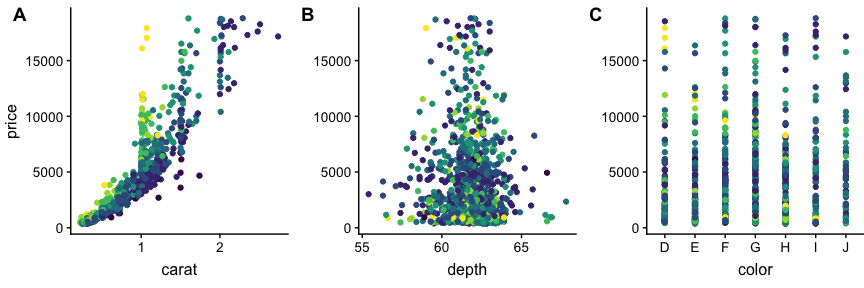Legend to the side:

# extract the legend from one of the plots
# (clearly the whole thing only makes sense if all plots
# have the same legend, so we can arbitrarily pick one.)
legend <- get_legend(p1)

# add the legend to the row we made earlier. Give it one-third of the width
# of one plot (via rel_widths).
p <- plot_grid( prow, legend, rel_widths = c(3, .3))
p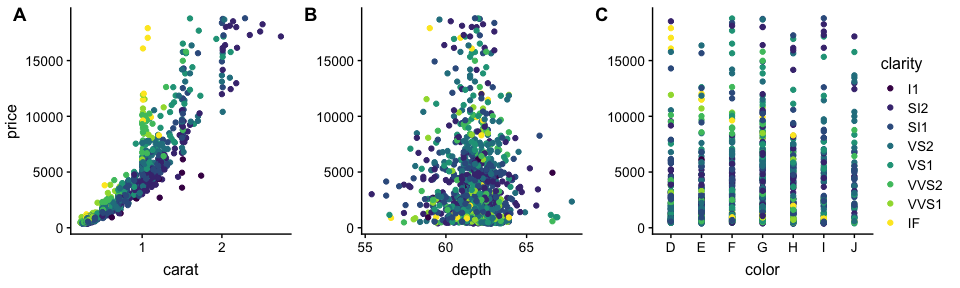Legend at the bottom:

# extract the legend from one of the plots
# (clearly the whole thing only makes sense if all plots
# have the same legend, so we can arbitrarily pick one.)
legend_b <- get_legend(p1 + theme(legend.position="bottom"))

# add the legend underneath the row we made earlier. Give it 10% of the height
# of one plot (via rel_heights).
p <- plot_grid( prow, legend_b, ncol = 1, rel_heights = c(1, .2))
p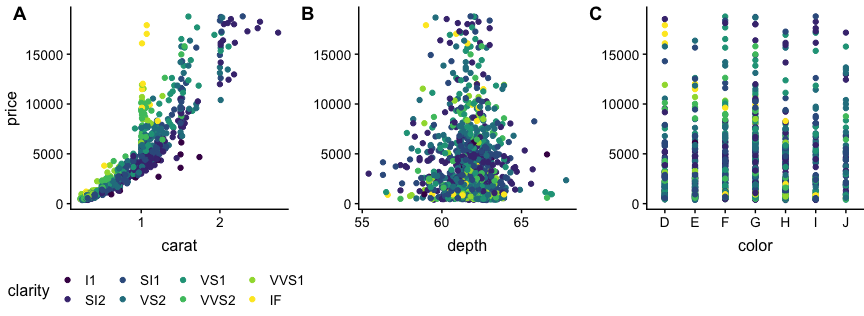Legend between plots:

# arrange the three plots in a single row, leaving space between plot B and C
prow <- plot_grid( p1 + theme(legend.position="none"),
p2 + theme(legend.position="none"),
NULL,
p3 + theme(legend.position="none"),
align = 'vh',
labels = c("A", "B", "", "C"),
hjust = -1,
nrow = 1,
rel_widths = c(1, 1, .3, 1)
)

prow + draw_grob(legend, 2/3.3, 0, .3/3.3, 1)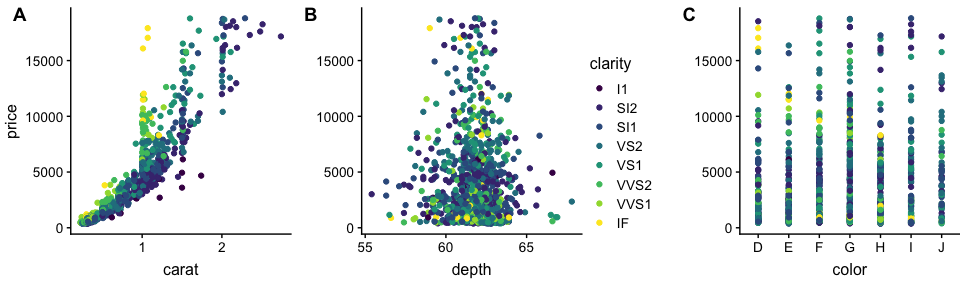One more example, now with a more complex plot arrangement:

# plot A
p1 <- ggplot(iris, aes(Sepal.Length, Sepal.Width, color = Species)) +
geom_point() + facet_grid(. ~ Species) + stat_smooth(method = "lm") +
background_grid(major = 'y', minor = "none") +
panel_border() + theme(legend.position = "none")

# plot B
p2 <- ggplot(iris, aes(Sepal.Length, fill = Species)) +
geom_density(alpha = .7) + theme(legend.justification = "top")
p2a <- p2 + theme(legend.position = "none")

# plot C
p3 <- ggplot(iris, aes(Sepal.Width, fill = Species)) +
geom_density(alpha = .7) + theme(legend.position = "none")

# legend
legend <- get_legend(p2)

# align all plots vertically
plots <- align_plots(p1, p2a, p3, align = 'v', axis = 'l')

# put together bottom row and then everything
bottom_row <- plot_grid(plots[], plots[], legend, labels = c("B", "C"), rel_widths = c(1, 1, .3), nrow = 1)
plot_grid(plots[], bottom_row, labels = c("A"), ncol = 1)